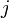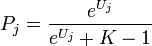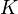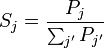Marketing - MaxDiff - Analyze as a Ranking Question - Compute Sawtooth-Style Preference Shares from Individual-Level Parameter Means (K Alternatives)

This QScript computes Sawtooth-style preference shares for the alternatives in a MaxDiff experiment using individual-level parameter means. The formula is given below.

Example

This example uses the data from the MaxDiff Case Study. The table on the left shows a SUMMARY of Individual-Level Parameter Means generated using Q's Segments tool. The table on the right shows a SUMMARY of the preference shares generated by this QScript. In this example there were 5 alternatives shown in each task, so a value of 5 was entered when the QScript was run.

Technical details

Input data

This QScript uses data from individual-level parameter means from a MaxDiff experiment. The variables should be combined into a Number - Multi question that has the string Individual-Level Parameter Means in the name. The individual-level parameters question can be generated in Q from a segmentation of a ranking question (by right-clicking a node of the tree and selecting Save Individual-Level Parameter Means and Standard Deviations).

Alternatively, the individual-level parameter means can be computed externally and imported into Q as part of a larger data set. In this case:

Outputs

A new Number - Multi question is generated in the project, and a table is added to the report to show the average of the computed values.

Preference-share formula

Each respondent's utilities are first zero-centered by subtracting the average. The zero-centered utility score for alternative$j$ is denoted$U_{j}$. The un-scaled Sawtooth-style preference parameter for alternative$j$ is then:$P_{j} = \frac{e^{U_{j}}}{e^{U_{j}} + K - 1}$

where$K$ is the number of alternatives that were shown to the respondent in each task of the MaxDiff experiment.

The final Sawtooth-style preference share is obtained by scaling these parameters so that they add up to 1 (or 100%):$S_{j} = \frac{P_{j}}{\sum_{j'}P_{j'}}$

How to apply this QScript

• Start typing the name of the QScript into the Search features and data box in the top right of the Q window.
• Click on the QScript when it appears in the QScripts and Rules section of the search results.

OR

• Select Automate > Browse Online Library.
• Select this QScript from the list.

Customizing the QScript

This QScript is written in JavaScript and can be customized by copying and modifying the JavaScript.

• Start typing the name of the QScript into the Search features and data box in the top right of the Q window.
• Hover your mouse over the QScript when it appears in the QScripts and Rules section of the search results.
• Press Edit a Copy (bottom-left corner of the preview).
• Modify the JavaScript (see QScripts for more detail on this).
• Either:
• Run the QScript, by pressing the blue triangle button.
• Save the QScript and run it at a later time, using Automate > Run QScript (Macro) from File.

Customizing QScripts in older versions

• Create a new text file, giving it a file extension of .QScript. See here for more information about how to do this.
• Modify the JavaScript (see QScripts for more detail on this).
• Run the file using Automate > Run QScript (Macro) from File.

JavaScript

includeWeb("QScript Functions for Setting Up Experiments");
var type = "Sawtooth-Style Preference Shares";
if (!maxDiffUtilityCalculation(type))
log("QScript Cancelled");
else
log("QScript Finished");